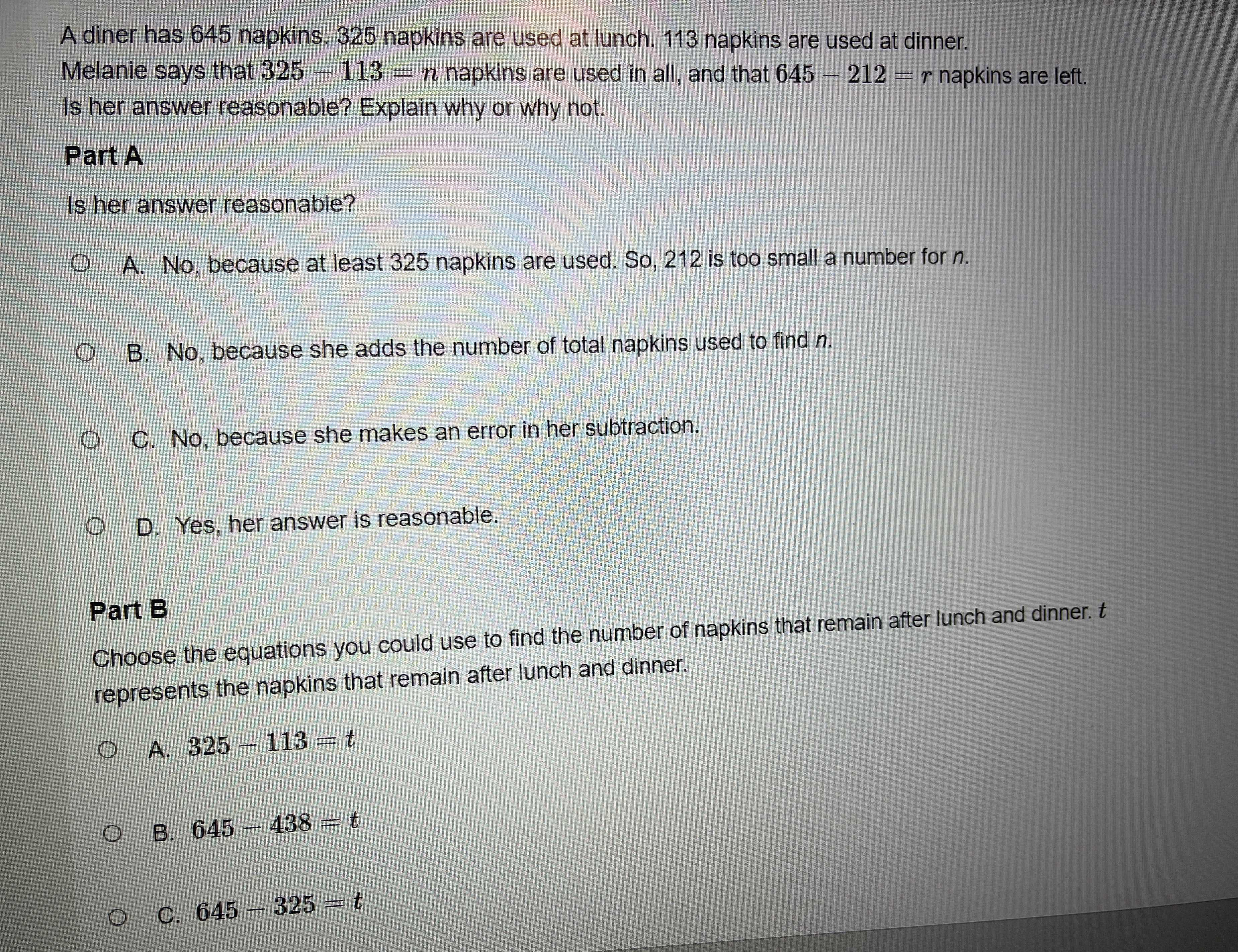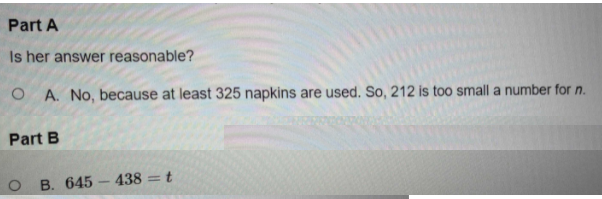### ¿Todavía tienes preguntas de matemáticas?

Pregunte a nuestros tutores expertos
Algebra
PreguntaA diner has $$645$$ napkins. $$325$$ napkins are used at lunch. $$113$$ napkins are used at dinner. Melanie says that $$325 - 113 = n$$ napkins are used in all, and that $$645 - 212 = r$$ napkins are left. Is her answer reasonable? Explain why or why not.

Part A

A. No, because at least $$325$$ napkins are used. So, $$212$$ is too small a number for $$n$$ .

B. No, because she adds the number of total napkins used to find $$n$$ .

C. No, because she makes an error in her subtraction.

D. Yes, her answer is reasonable.

Part $$B$$

Choose the equations you could use to find the number of napkins that remain after lunch and dinner. $$t$$ represents the napkins that remain after lunch and dinner.

A. $$325 - 113 = t$$

B. $$645 - 438 = t$$

C. $$645 - 325 = t$$